# Scientific Notation Scientific Notation is a compact way

• Slides: 25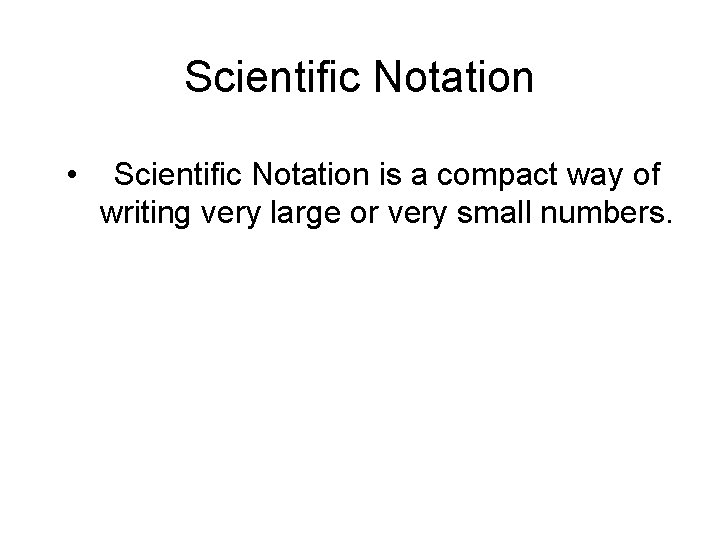Scientific Notation • Scientific Notation is a compact way of writing very large or very small numbers.A number is expressed in scientific notation when it is written as the product of a factor and a power of 10. Factor Power of 10 The factor must be greater than or equal to 1 and less than 10.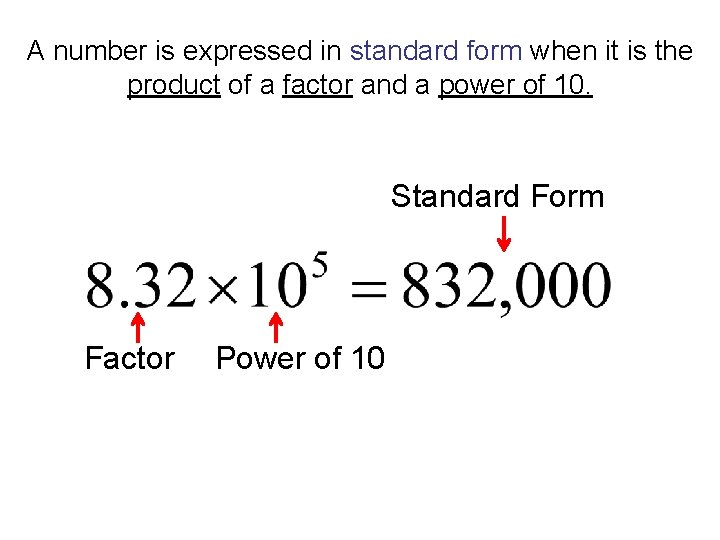A number is expressed in standard form when it is the product of a factor and a power of 10. Standard Form Factor Power of 10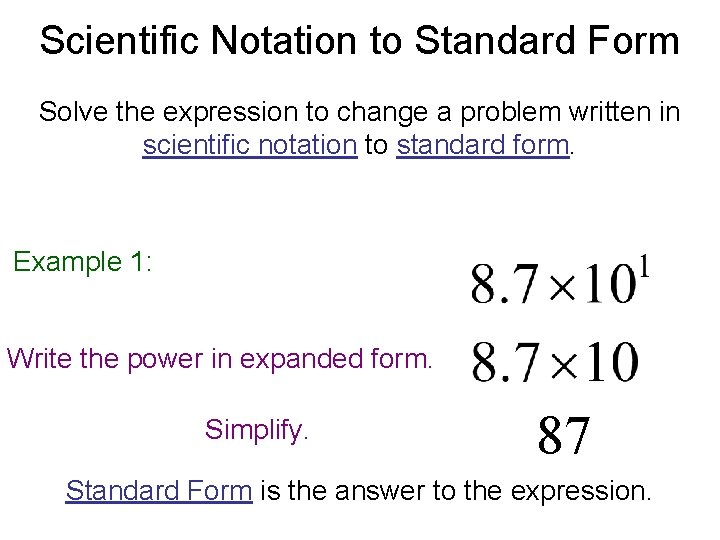Scientific Notation to Standard Form Solve the expression to change a problem written in scientific notation to standard form. Example 1: Write the power in expanded form. Simplify. 87 Standard Form is the answer to the expression.Scientific Notation to Standard Form Solve the expression to change a problem written in scientific notation to standard form. Example 2: Write the power in expanded form. Simplify. 870 Standard Form is the answer to the expression.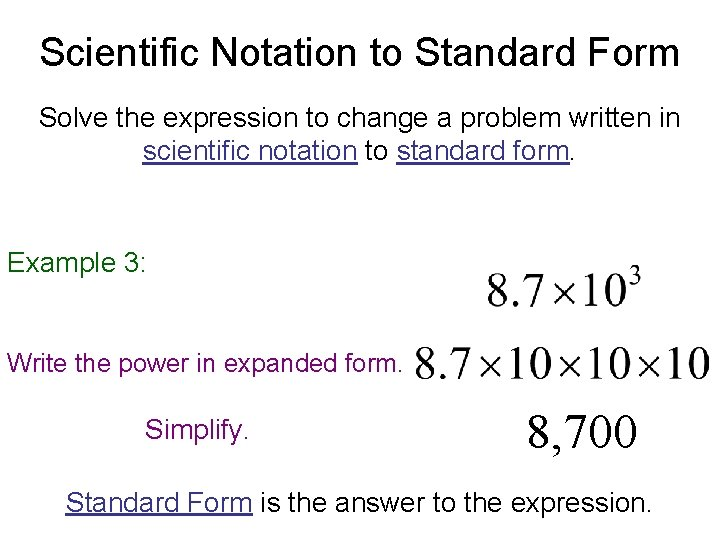Scientific Notation to Standard Form Solve the expression to change a problem written in scientific notation to standard form. Example 3: Write the power in expanded form. Simplify. 8, 700 Standard Form is the answer to the expression.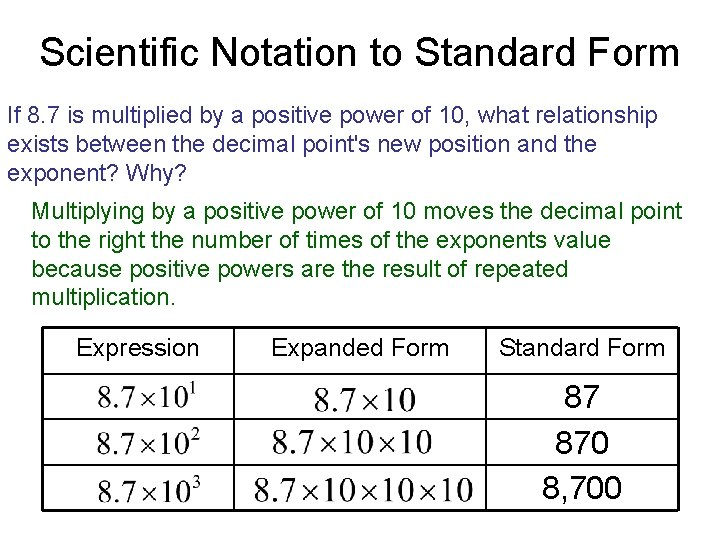Scientific Notation to Standard Form If 8. 7 is multiplied by a positive power of 10, what relationship exists between the decimal point's new position and the exponent? Why? Multiplying by a positive power of 10 moves the decimal point to the right the number of times of the exponents value because positive powers are the result of repeated multiplication. Expression Expanded Form Standard Form 87 870 8, 700Scientific Notation to Standard Form Solve the expression to change a problem written in scientific notation to standard form. Example 4: Write the power in expanded form. Simplify. 0. 87 Standard Form is the answer to the expression.Scientific Notation to Standard Form Solve the expression to change a problem written in scientific notation to standard form. Example 5: Write the power in expanded form. Simplify. 0. 087 Standard Form is the answer to the expression.Scientific Notation to Standard Form Solve the expression to change a problem written in scientific notation to standard form. Example 6: Write the power in expanded form. Simplify. 0. 0087 Standard Form is the answer to the expression.Scientific Notation to Standard Form If 8. 7 is multiplied by a negative power of 10, what relationship exists between the decimal point's new position and the exponent? Why? Multiplying by a negative power of 10 moves the decimal point to the left the number of times of the exponents value because negative powers are the result of repeated division. Expression Expanded Form Standard Form . 87. 0087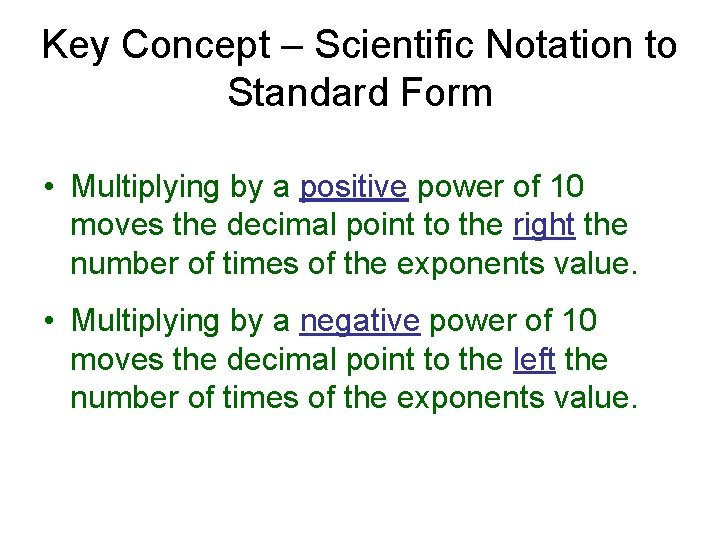Key Concept – Scientific Notation to Standard Form • Multiplying by a positive power of 10 moves the decimal point to the right the number of times of the exponents value. • Multiplying by a negative power of 10 moves the decimal point to the left the number of times of the exponents value.Ex: Write 5. 34 x 104 in standard form. Method 1: 1. Write the power in expanded form. 53, 400 2. Simplify. Method 2: OR 1. Determine how many places the decimal is going to move. 2. Determine which direction the decimal is going to move. 5 3 40 0 1 2 3 4 53, 400Ex: Write 3. 27 x 10 -3 in standard form. Method 1: 1. Write the power in expanded form. 2. Simplify. Method 2: 0. 00327 OR 1. Determine how many places the decimal is going to move. 2. Determine which direction the decimal is going to move. 003 2 7 3 2 1 0. 00327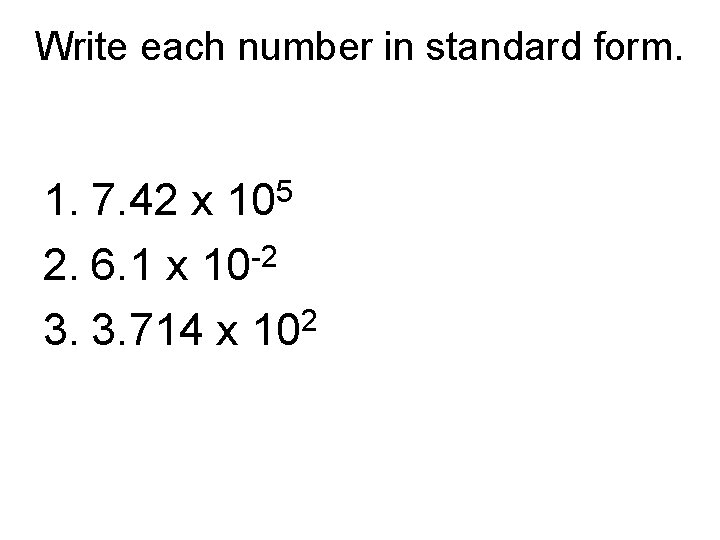Write each number in standard form. 1. 7. 42 x 105 -2 2. 6. 1 x 10 2 3. 3. 714 x 10Standard Form to Scientific Notation To write a number in scientific notation, follow these steps. 1. Move the decimal point to the right of the first nonzero digit. 2. Count the number of places you moved the decimal point. 3. Find the power of 10. If the original number was between 0 and 1, the exponent is negative. Otherwise, the exponent is positive.Write 3, 725, 000 in scientific notation Step 1: Move the decimal point to the right of the first nonzero digit. 3, 725, 000 Why do you need to move the decimal to the right of the first nonzero digit? Moving the decimal to the right of the first nonzero number finds the factor part of the scientific notation. What is the factor? 3. 725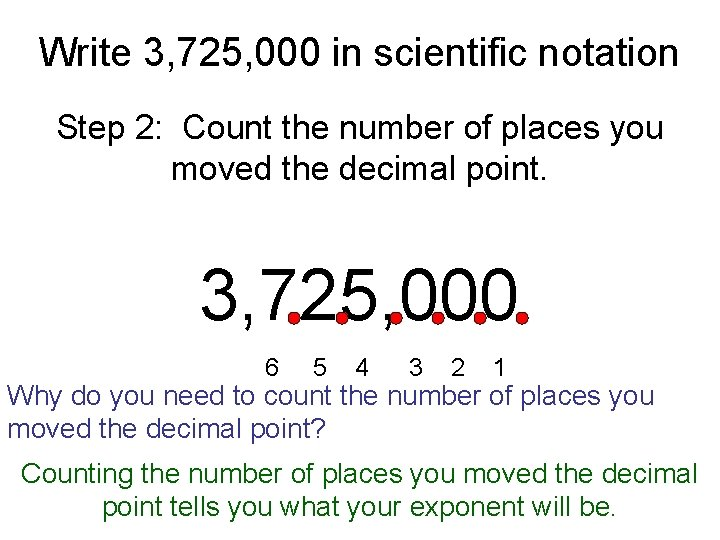Write 3, 725, 000 in scientific notation Step 2: Count the number of places you moved the decimal point. 3, 725, 000 6 5 4 3 2 1 Why do you need to count the number of places you moved the decimal point? Counting the number of places you moved the decimal point tells you what your exponent will be.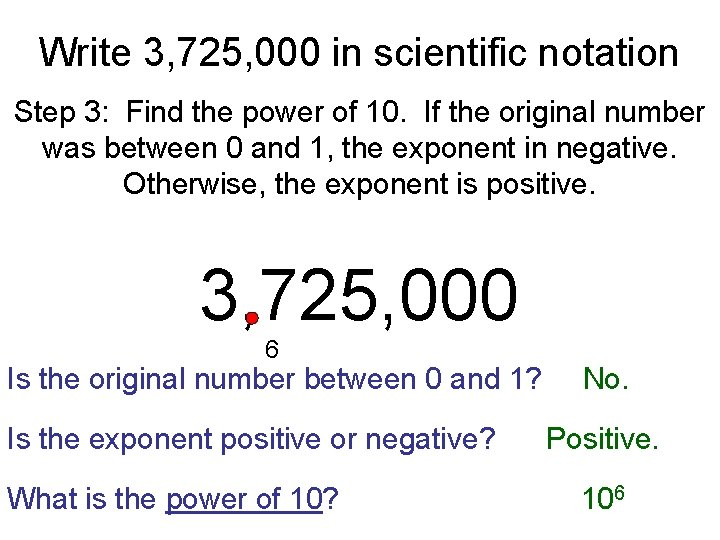Write 3, 725, 000 in scientific notation Step 3: Find the power of 10. If the original number was between 0 and 1, the exponent in negative. Otherwise, the exponent is positive. 3, 725, 000 6 Is the original number between 0 and 1? Is the exponent positive or negative? What is the power of 10? No. Positive. 106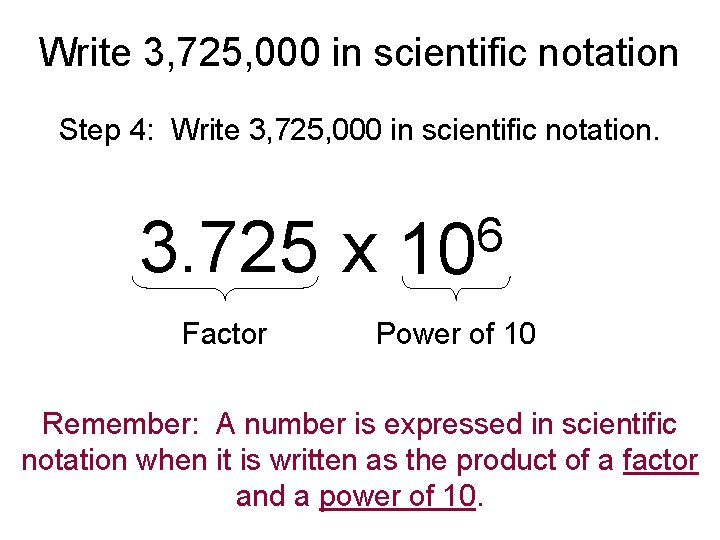Write 3, 725, 000 in scientific notation Step 4: Write 3, 725, 000 in scientific notation. 3. 725 x Factor 6 10 Power of 10 Remember: A number is expressed in scientific notation when it is written as the product of a factor and a power of 10.Write 0. 000316 in scientific notation Step 1: Move the decimal point to the right of the first nonzero digit. 0. 000316 Why do you need to move the decimal to the right of the first nonzero digit? Moving the decimal to the right of the first nonzero number finds the factor part of the scientific notation. What is the factor? 3. 16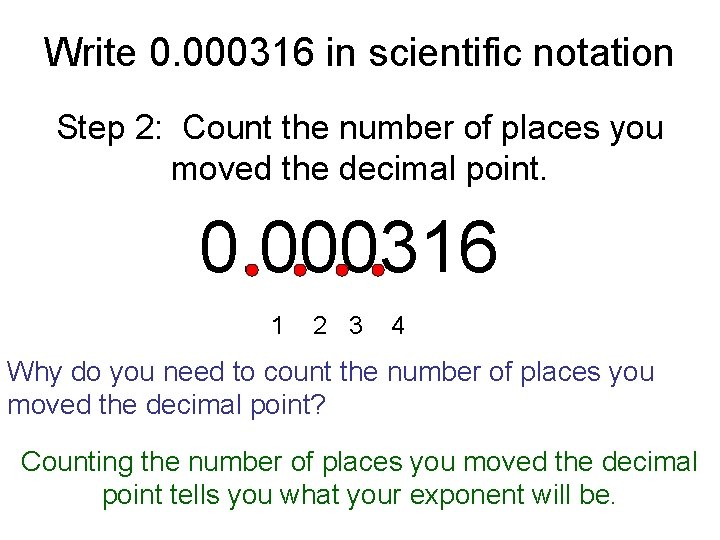Write 0. 000316 in scientific notation Step 2: Count the number of places you moved the decimal point. 0. 000316 1 2 3 4 Why do you need to count the number of places you moved the decimal point? Counting the number of places you moved the decimal point tells you what your exponent will be.Write 0. 000316 in scientific notation Step 3: Find the power of 10. If the original number was between 0 and 1, the exponent is negative. Otherwise, the exponent is positive. 0. 000316 4 Is the original number between 0 and 1? Is the exponent positive or negative? What is the power of 10? Yes. Negative. 10 -4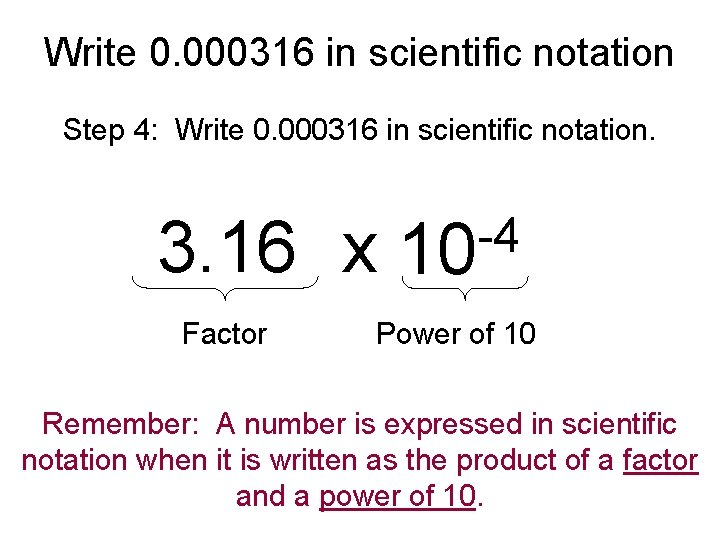Write 0. 000316 in scientific notation Step 4: Write 0. 000316 in scientific notation. 3. 16 x Factor -4 10 Power of 10 Remember: A number is expressed in scientific notation when it is written as the product of a factor and a power of 10.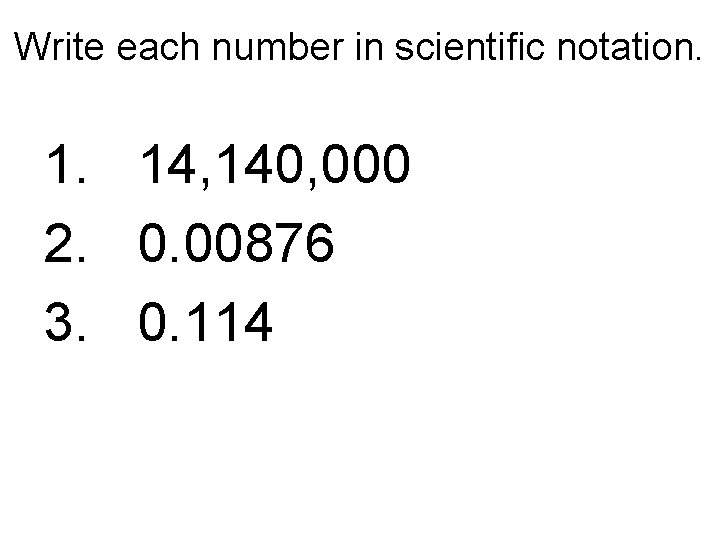Write each number in scientific notation. 1. 14, 140, 000 2. 0. 00876 3. 0. 114